# Rotations of order 6 with reflections

The remaining patterns are numbered 519, which implies that one more further question needs to the answered.

The first group are examples of patterns with a hexagon. An example of two of these are: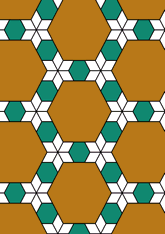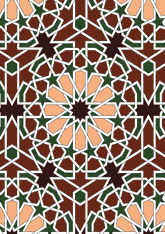The number in this group is 187, which is 36% of the total. Another question is needed - for this see page.
The second group are examples of patterns which have a 6-pointed star (ignoring ones with diamonds), but not a hexagon. An example of two of these are: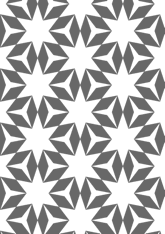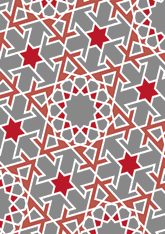The number in this group is 189, which is 36% of the total. Another question is needed - for this see page.
The last group are examples of patterns with diamonds. An example of two of these are: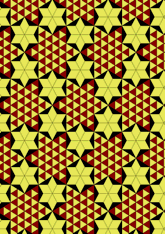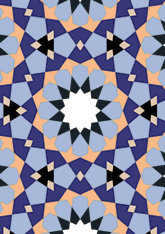The number in this group is 31, which is 5% of the total. Another question is needed - for this see page.
The last group are examples of patterns which have neither a hexagon or a 6-pointed star. An example of two of these are: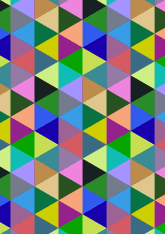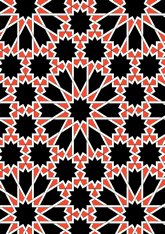The number in this group is 112, which is 21% of the total. Another question is needed - for this see page.

v53

Tiling Search was created by Brian Wichmann, and is maintained by MIT Libraries, through support from the Aga Khan Documentation Center.

Also supported by Performant Software Solutions LLC.